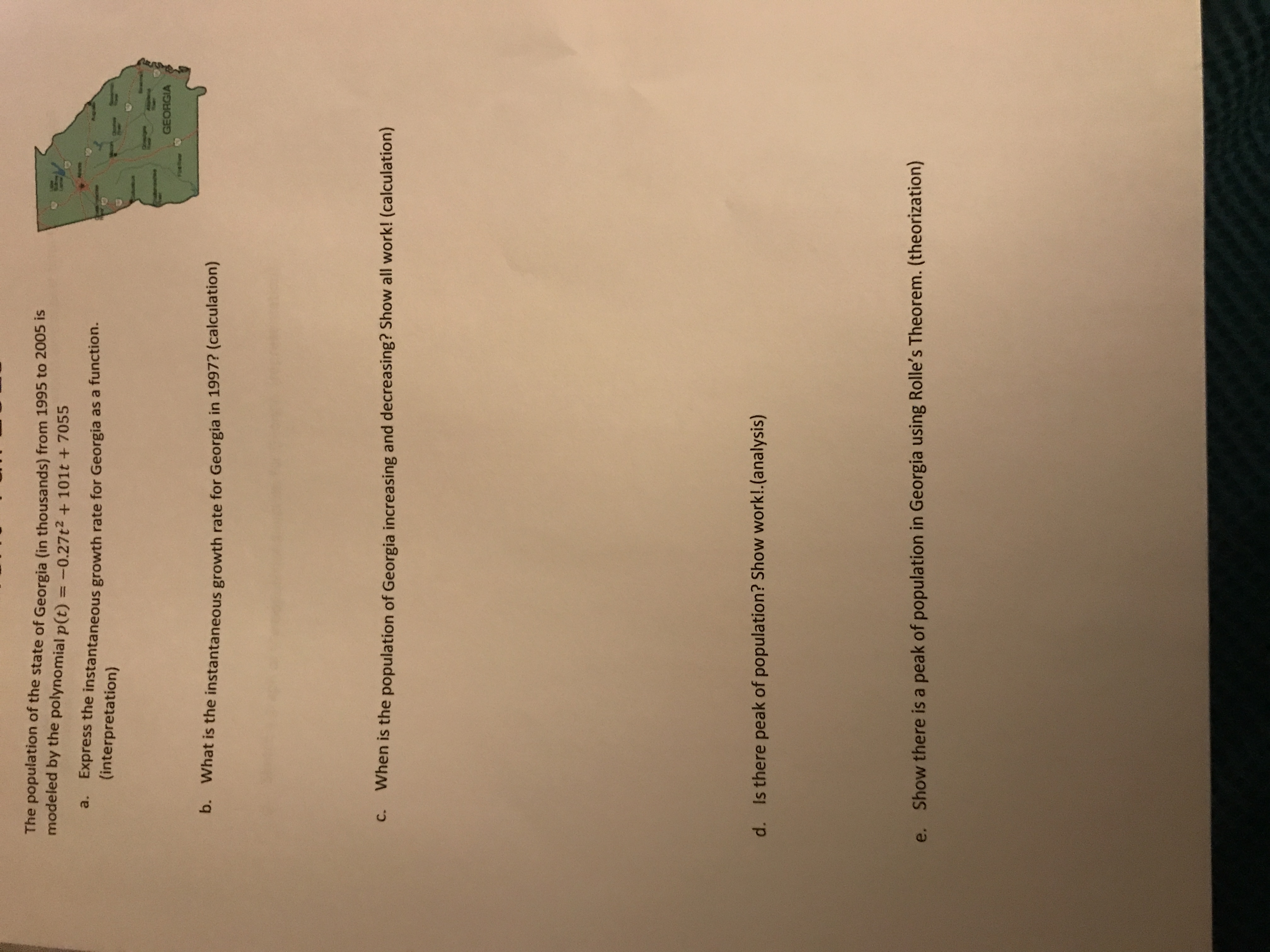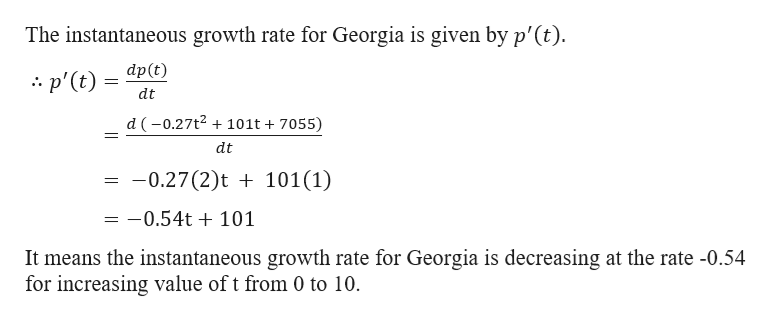# The population of the state of Georgia (in thousands) from 1995 to 2005 ismodeled by the polynomial p(t) =-0.27t2 + 101t + 7055Aa.Express the instantaneous growth rate for Georgia as a function.(interpretation)OoslgGEORGIAFeurb. What is the instantaneous growth rate for Georgia in 1997? (calculation)C.When is the population of Georgia increasing and decreasing? Show all work! (calculation)Is there peak of population? Show work!.(analysis)d.e. Show there is a peak of population in Georgia using Rolle's Theorem. (theorization)

Question
39 viewshelp_outlineImage TranscriptioncloseThe population of the state of Georgia (in thousands) from 1995 to 2005 is modeled by the polynomial p(t) =-0.27t2 + 101t + 7055 A a. Express the instantaneous growth rate for Georgia as a function. (interpretation) Ooslg GEORGIA Feur b. What is the instantaneous growth rate for Georgia in 1997? (calculation) C. When is the population of Georgia increasing and decreasing? Show all work! (calculation) Is there peak of population? Show work!.(analysis) d. e. Show there is a peak of population in Georgia using Rolle's Theorem. (theorization) fullscreen
check_circle

Step 1

Given,

The population of the state of Georgia (in thousands) from 1995 to 2005 is modelled by the polynomial p(t) = -0.27t2 + 101t + 7055.help_outlineImage TranscriptioncloseThe instantaneous growth rate for Georgia is given by p'(t). dp(t) p'(t) dt d (0.27t2101t + 7055) dt 0.27(2)t101(1) 0.54t 101 It means the instantaneous growth rate for Georgia is decreasing at the rate -0.54 for increasing value of t from 0 to 10 fullscreen
Step 2

Part b

Taking 1995 as t = 0 then

For 1997, t = 1997...

### Want to see the full answer?

See Solution

#### Want to see this answer and more?

Solutions are written by subject experts who are available 24/7. Questions are typically answered within 1 hour.*

See Solution
*Response times may vary by subject and question.
Tagged in

### Derivative